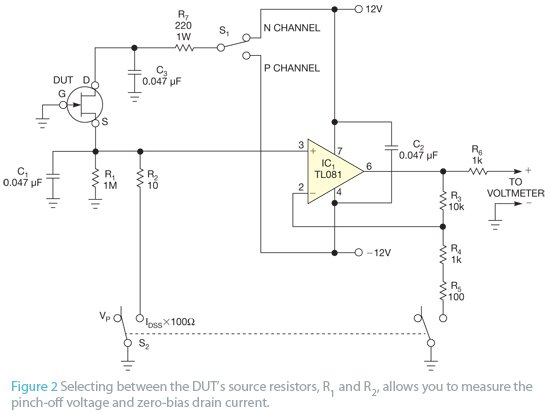# Simple circuit lets you characterize JFETs

When working with discrete JFETs, designers may need to accommodate a large variation in device parameters for a given transistor type. A square-law equation is usually used as an approximate model for the drain-current characteristic of the JFET: ID=β(VGS−VP)2, where ID is the drain current, VGS is the gate-to-source voltage, β is the transconductance parameter, and VP is the gate pinch-off voltage. With this approximation, the following equation yields the zero-bias drain current at a gate-to-source voltage of 0V: IDSS=βVP 2, where IDSS is the zero-bias drain current.Figure 1 is a plot of this characteristic for N-channel JFETs showing the variation possible in a collection of devices. For example, the 2N4416A’s data sheet lists a pinch-off voltage of −2.5 to −6V, and the zero-bias drain current can range from 5 to 15 mA. You can observe the correlation between these two parameters across a sample of devices. The outer curves in the plot represent these extreme cases, and the center curve represents perhaps a typical case of a pinchoff voltage of −4V and a zero-bias drain current of 8 mA.

Although you can design around a certain amount of device variation for a mass-produced circuit, you sometimes need a tool to quickly characterize an assortment of discrete devices. This tool allows you to select a device that will optimize one circuit or perhaps to find a pair of devices with parameters that match reasonably well.

Figure 2 shows a simple test circuit for this purpose. Although the figure shows the JFET as an N-channel device, the JFET DUT (device under test) may be of either polarity, as selected by switch S1. An external voltmeter connects to the terminals on the right. Switch S2 selects two distinct measurement modes—one for the pinch-off voltage and another for the zero-bias drain current. In the pinch-off-voltage mode, the external voltmeter directly reads the pinch-off voltage; in the zero-bias-drain-current mode, the measured voltage is the zero-bias drain current across an apparent resistance of 100Ω.

READ  Cliff knobs - a pleasure to touch

With S2 in the pinch-off-voltage mode, R1 allows a few microamps of drain current to flow in the JFET under test, and the source voltage is a close approximation of the negative of the pinch-off voltage. The op amp acts as a unity-gain buffer, with negative feedback through R3, so you can directly read the negative of the pinch-off voltage with the external voltmeter.
In the zero-bias-drain-current mode, however, the resistance from JFET source to ground is only 10Ω, so the drain current is a close approximation of the zero-bias drain current. The op amp’s feedback also switches to a gain-of-10 configuration, with the inclusion of R4 and R5 in the feedback-voltage divider. This gain allows the voltmeter to easily read the small voltage across R2, with the resulting reading being the zero-bias drain current times 100Ω. For example, if the voltmeter reads 1V, this voltage corresponds to a zero-bias drain current of 10 mA.

For an N-channel device, both voltage readings are positive; for a P-channel device, the circuit functions in the same manner except that the voltage readings are negative. If you wire the test JFET to this circuit with test leads and clips, each with some parasitic series inductance, you may need to add C1 to suppress any tendency for high-frequency oscillation. R6 isolates the op-amp feedback loop from any parasitic capacitance in the voltmeter and its leads, preserving the loop stability. R7 protects against accidental shorts, and you can replace R4 and R5 with one 1.1-kΩ resistor. You are more likely to have on hand resistors with the values in the figure, however.

By clipping in samples from a collection of JFETs and throwing a switch, you can very quickly find the two parameters that determine where each JFET’s characteristic falls in the range that Figure 1 illustrates and select devices to optimize circuit performance.

READ  MintBox Mini 2 comes with more power and it is 50% faster than its predecessor.

For more detail: Simple circuit lets you characterize JFETs